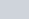If A and B are (1, 4) and (5, 2) respectively,
Question:

If A and B are (1, 4) and (5, 2) respectively, find the coordinates of P when AP/BP = 3/4.

Solution:

The co-ordinates of the point dividing two points $\left(x_{1}, y_{1}\right)$ and $\left(x_{2}, y_{2}\right)$ in the ratio $m: n$ is given as,

$(x, y)=\left(\left(\frac{\lambda x_{2}+x_{1}}{\lambda+1}\right),\left(\frac{\lambda y_{2}+y_{1}}{\lambda+1}\right)\right)$ where, $\lambda=\frac{m}{n}$

Here the two given points are A(1,4) and B(5,2). Let point P(x, y) divide the line joining ‘AB’ in the ratioSubstituting these values in the earlier mentioned formula we have,

$(x, y)=\left(\left(\frac{\frac{3}{4}(5)+(1)}{\frac{3}{4}+1}\right),\left(\frac{\frac{3}{4}(2)+(4)}{\frac{3}{4}+1}\right)\right)$

$\left.(x, y)=\left(\frac{\frac{15+4(1)}{4}}{\frac{3+4}{4}}\right),\left(\frac{\frac{6+4(4)}{4}}{\frac{3+4}{4}}\right)\right)$

$(x, y)=\left(\left(\frac{19}{7}\right),\left(\frac{22}{7}\right)\right)$

Thus the co-ordinates of the point which divides the given points in the required ratio are $\left(\frac{19}{7}, \frac{22}{7}\right)$.